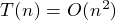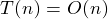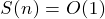## Bubble Sort Back

• 冒泡排序 :每趟都把最大的沉到后。
• 时间复杂度：
•(最壞平均情況)
•(最好情況)
• 空間複雜度：• 稳定性 :稳定
• 适用情况 :数据少, 初始序列基本有序
``````void BUBBLE_SORT(int num[], int size)
{
for (int i = 0; i < size; i++)
{
for (int j = 0; j < size - i; j++)
{
if (num[j] > num[j + 1])
{
int temp = num[j];
nun[j] = num[j + 1];
num[j + 1] = temp;
}
}
}
}
``````